# Oberseminar Analysis Winter 2018/19

## Organizers: S. Conti, H. Koch, S. Müller, B. Niethammer, M. Rumpf, C. Thiele, J.J.L. Velázquez, R. Winter

• Thursday October 25 2:15 p.m., Lipschitz-Saal
Amrita Gosh (University of Pau)
Navier-Stokes equations with Navier boundary condition and behaviour with respect to the slip length
Consider the dynamics of a viscuous incompressible fluid given by the non-stationary Navier Stokes equation with slip boundary condition in a bounded domain \begin{equation} \begin{aligned} \frac{\partial u}{\partial t} - \Delta u + (u\cdot \nabla )u + \nabla \Pi &=0, && \text{in $\Omega \times (0,T)$} \\ u \cdot n=0, \quad 2 [(\mathbb{D} u)n]_\tau + \alpha u_\tau &=0, && \text{in $\Gamma \times (0,T)$} \\ u(0)&=u_0, && \text{in $\Omega$} . \end{aligned} \end{equation} Here $\Omega$ is a bounded domain in $\mathbb{R}^3$ with boundary $\Gamma$. The initial velocity $u_0$ and the (scalar) friction coefficient $\alpha$ are given functions. The unknowns are $u$ and $\Pi$ which describe respectively the velocity and the pressure of the fluid flow. The boundary condition in (1) was introduced by H. Navier which is in recent years widely studied because of its significance in different real world models for simulation of flows and fluid-solid interaction problems. I will discuss the well-posedness of the above system imposing minimal regularity on $\alpha$. Weak and strong solutions of the associated Stokes operator and the resolvent estimate, uniform in the friction coefficient $\alpha$ is deduced which enables us to obtain bounds on the solution of (1) independent of $\alpha$. Finally, we study the behaviour of the solution of (1) with respect to the friction coefficient, in particular what happens if $\alpha$ goes to $\infty$.

• Thursday January 24 2:15 p.m., Lipschitz-Saal
Johanna Burtscheidt (Universität Duisburg-Essen)
Bilevel Problems and Stability Results for a Risk Averse Linear Bilevel Problem under Stochastic Uncertainty
Bilevel optimization problems arise from the interplay between two decision makers on different levels of a hierarchy. In stochastic bilevel problems, the lower level problem is entered by the realization of some random vector whose distribution does not depend on the upper level decision as an additional parameter. It is assumed that the leader has to make his or her decision without knowing the realization of the randomness, while the follower decides under full information. Linear two-stage stochastic programs form a subclass of linear stochastic bilevel problems. In addition, we get weaker analytical properties for bilevel problems compared to two-stage problems. We examine linear bilevel problems, where the right-hand side of the lower level problem is subject to stochastic uncertainty, and present a risk averse formulation based on special risk measures. In particular, structural properties and qualitative stability of the optimal value function of this model under perturbation of the underlying Borel probability measure will be investigated with respect to weak convergence of probability measures. Focussing on the case of an underlying random vector with a finite number of realizations, equivalences of the risk averse stochastic bilevel problems and single-level problems conclude the talk. This is joint work with Matthias Claus and Stephan Dempe.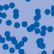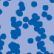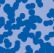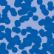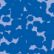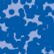## News

Florian Schweiger erhielt den Hausdorff-Gedächtnispreis 2021 der Fachgruppe Mathematik für die beste Dissertation. Er fertigte die Dissertation unter der Betreuung von Prof. Stefan Müller an. Unter anderen wurde Vanessa Ryborz mit einem Preis der Bonner Mathematischen Gesellschaft für ihre von Prof. Sergio Conti betreute Bachelorarbeit ausgezeichnet. (18.01.2022)

Prof. Dr. Sergio Albeverio has been elected into the Academia Europaea and the Accademia Nazionale dei Lincei (more; 02.12.2021).

Der SFB 1060 Die Mathematik der emergenten Effekte hat eine dritte Förderperiode erhalten. (26.11.20)

Prof. Dr. Andreas Eberle erhält den diesjährigen Lehrpreis der Universität Bonn. (22.07.2020)

Herr Dr. Richard Höfer erhielt den Hausdorff-Gedächtnispreis 2019 der Fachgruppe Mathematik für die beste Disseration. Betreut wurde die Arbeit von Prof. J. Velázquez (29.01.2020).

Contact

Managing Director: Prof. Dr. Massimiliano Gubinelli